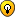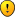## Incredibly difficult kakuro (14x14)

For fans of Kakuro

### Incredibly difficult kakuro (14x14)

I spent a few days making this puzzle (especially the lower right) trying to keep the solution unique and hard to find:Here is the Janko java-applet form:
http://www.h3lixmusic.com/kakuro/ka2.html

Good luck.
h3lix

Posts: 8
Joined: 04 December 2006

It's too bad not many have noticed this great kakuro puzzle... If you're a kakuro lover I'm sure you'd enjoy this one!

A hint for those who are challenged:

From this pic, you can deduce the sums of a couple groups of cells,
which will come very useful during the solving process!Sum of yellow solid cells (r7c4+r8c34)
= (24+15+11+34+20+8 ) - (8+11+27+6+21+8+11)
= 112 - 92
= 20

Sum of green solid cells (r6cAB+r7cA)
= (6+16+40+26+18+26) - (13+15+21+13+19+29+12)
= 132 - 122
= 10

And here is my complete walkthrough:

I did it in quite a neat sequence... The grid felt like a 4-leaf clover, where I paint the leaves up one by one...

Notation: Rows/columns have indices as 1..9,A,B,C,D (although it's tempting to use A,2..9,T,J,Q,K!)

Useful combinations:
23/6={1234(58|67)}
37/6={(25|34)6789}
34/7={123456789}-{29|38|47|56}
40/7={(14|23)56789}

Stage 1:
r12c2=4/2= (r2c2 @ 37/6 cannot be 1)
r12c1=6/2= (37/6 @ r2c1..6 must be {346789})
r12c3=9/2= (r2c3 cannot be 7,8,9)
=> r1c456 @ 23/6={4(58|67)}, r2c456 @ 37/6={789}
r1234c5=14/4={12345678} => r2c5=7|8
r134c5=14-7|8=6|7={123|124} => r12c5=, r34c5={12}
=> r1c46={58|67}, r2c46={89}
r12c4 @ 19/5 cannot exceed 13 (min r345c4={123}=6)
=> r12c4=, r345c4={123}, r123c6=
r3c45 @ 17/4 cannot be {12}=3 (max r3c67={49}=13)
=> r3c4=3, r3c57=[19|28], r45c4={12}
r4c123=25-3=22={(58|67)9}, r456c3=8/3={1(25|34)}
=> r4c3=5, r4c12={89}, r56c3={12}
r5c12=19-3=16={79}
r6c12=16-1|2=14|15={56789}
r456c2 @ 26/5 cannot be {789}=24 (min r78c2={12}=3)
=> r6c2=5|6
r6c1 @ 16/3 must exceed 7 (max r6c23==8 )
=> r6c1=8|9
r46c1={89} => r5c12= => r4c12=, r6c123=
=> r5c34=, r4c45=, r3c57=
r7c12=3/2={12}, r78c2=26-23=3={12}, r78c1=29-24=5=[14|23]

Stage 2:
r4c78=15/2=[69|78|87], r45c8=14/2={59|68}
=> r4c78=[69|78], r45c8=[86|95]
r5c67=20-5|6=14|15={56789}, while r567c6=10/3={1234567}
r5c68 @ 20/3 cannot be {56}=11 (max r5c7=8 )
=> r5c6=7, r67c6={12}, r5c78=20-7=13=, r4c78=
r6c57=17-1|2=15|16=[87|97], while r678c5=11/3={12345678}
=> r6c567=, r7c6=1, r78c5=, r78c2=, r78c1=
r89ABc3=11/4={1235} => r8c34=18-6=12=, r9c34=8/2=[26|35]
rAc1234=11/4={1235}
rABc12=24+15-6-8=25 => rAc12={35}, rBc12={89}, rAc34={12}
rABCDc2=15/4={1239|1248} cannot be {1248} (rAc2=3|5)
=> rABc2=, rCDc2={12}, rABc1=
=> rCc12=6/2=, rDc12=8/2=
r7c4+r8c34=20 (sum of yellow solid cells) => r7c4=20-5-7=8
{38},{47},{56} must stay in r7..Dc4 => r7..Dc4=34/7={1345678}
=> rAc34=, r9c34=, rBc3=1, rBCDc4={346}
rCc456=21/3={456789}, rCDc6=8/2=[53|62|71]
rCc4 @ 21/3 must exceed 4 (max rCc56==16)
=> rCc4=6, rCc56=21-6=15=, rDc6=1, rBDc4={34}
rBc45=27-18=9=[36|45], rDc45=11-1=10=[37|46]
rBDc5=20-8=12=, rBDc4=

Stage 3:
r789ABc7={12345}, r789c8=21/3={456789}
rAc67=8/2={17|26|35}, r9Ac6=12/2={39|48|57}
r9c6 @ 11/3 cannot exceed 6 (min r9c78==5)
=> r9Ac6=, rAc7=1, r9c78=11-5=6=, r78c8=21-4=17=
r678c9=20/3={3456789} => r8c79=15-8=7={34}
r67c9=20-3|4=16|17={79|89}, while r7c9 cannot be 8,9
=> r678c9=, r8c7=3, r7Bc7={45}, r7c7A=36-27=9={45}
r6cAB+r7cA=10 (sum of green solid cells) => r6cAB=10-4|5=5|6
r6cAB @ 30/5 must exceed 5 (max r6c9CD={789}=24)
=> r6cAB=6={15|24}, r7cA=4, r7Bc7=, r6cCD={78}
r3456cB=26/4={23456789} => r6cAB=[15|24]
r1234567cA=40/7={(14|23)56789} => r67cA cannot be 
=> r6cAB=, r12345cA={56789}
r4cABCD=29/4={5789}
r34cCD=18+26-15-13=16 => r4cCD={57|58}, r3cCD={12|13}
r1234cD=26/4={23456789} => r3cC=1, r3cD=2|3
r4cD @ 26/4 cannot be 5 (max r123cD={389}=20)
=> r4cC=5, r34cD=16-6=10=[28|37]
r1cCD=15/2={69|78}, r2cCD=13/2={49|58|67}
r12cC=18-6=12= => r1234cD=, r4cAB={79}
r345cB=26-5=21={(49|67)8}, while r5cAB=12/2 cannot be 
=> r345cB= (r5cB @ 12/2 cannot be 6), r45cA=
r3c9A=19-11=8=, r12cA={69}
r2c89A=21/3={456789} => r12c8=6/2=[15|24]
r2cA @ 21/3 cannot be 6 (max r2c89==14) => r12cA=
r1c89=13-6=7=, r2c89=

Stage 4:
r7cCD=16/2={79}
r67cC @ 21/5 cannot be =16 (min r89AcC={123}=6)
=> r67cC=, r67cD=, r89AcC={123}, r89AcD=23-16=7={124}
r8cD @ 15/3 must exceed 2 (max r8cBC==12)
=> r8cD=4, r9AcD={12}
rAcCD @ 28/5 cannot be {12}=3 (max rAc9AB={789}=24)
=> rAcC=3, r89cC={12}
r8cBC=15-4=11= => r9cC=1, r9AcD=, r9AcB=24-9=15={78}
r9cAB=15-3=12=[48|57], rAc9AB=28-4=24={789}
rCDcB=14/2={59|68}, rCDcD=15/2={69|78}
rDc89ABCD=23/6={1234(58|67)} => rDc89AC={1234}, rDcBD=[58|67]=13
rCcBD=14+15-13=16= => rDcBD=
rCDcC=11/2= (rDcC cannot be 1,2,4)
=> rCc89A @ 37/6={(25|34)6}, rDc89A @ 23/6={124}
rDcA @ 32/5 must exceed 2 (max r9ABCcA={5689}=28 )
=> rDcA=4, r9cAB=, rAcB=8, r9ABCDcA=32/5=, rAc8=7
rDc89={12}, rCc89={25|34}
rBCDc8=7/3={124} => rCc89=, rBDc8={12}
rBc89=20-12=8= => rDc89=

And finally, here is the solution...udosuk

Posts: 2698
Joined: 17 July 2005

Hi h3lix,

What a GREAT puzzleIt took HOURS of PAINSTAKING effort, a box of pencils, a supply of erasers and a reem of scribbling paper; but I EVENTUALLY solved it. Ran out of midnight oil, though!!

Your puzzle ranks right up there with the most enjoyable logic puzzles that I've ever done (my favourites are a couple of real teasers: a mathematical cross-number puzzle called "Little Piggly Farm", aka "Dogs Mead; and "Self-Referential Aptitude Test", by Jim Propp).

It is VERY craftily constructed, with hard-to-spot threads and the use of clues containing either the most or at least a great many combinations. And there was an interesting hurdle to jump where I got stuck. Right the way through, especially at the beginning, achieving completion appears to be a very daunting task - GREAT!

For me, one of the most attractive features of your puzzle is the personal, rather than computer-generated, construction. Of course, computers can't generate anything without human input, but it gave the whole exercise quite a different flavour (akin to doing a cryptic cross-word puzzle, where the composer has gone out of his way to create something full of red herrings and stumbling blocks to make life difficult for the would-be solver).

FYI, I entered the puzzle into Indigo Puzzles' solver at one stage, hoping that their Assistant could help me negotiate the impasse that I mentioned before. However, the solver rejected the puzzle. So did KakuroWorks, which said that it had more than one solution (untrue - there is only one).

Now to check out udosuk's walkthrough to see how I SHOULD have done it, and how I could have avoided fumbling around and grinding away endlessly with countless sweepings and winnowings.

Anyway, I loved it. Congratulations!

Got any more?Surfertje

Posts: 14
Joined: 15 May 2007

Here is another hint I posted on another forum about the differences between row/column

This could also be extended to get difference of cells, similar to innies & outies of killer as in this picture:

At the top
r12cA (yellow) - r3c9 (blue) = (13+21) - (6+16) = 12
-> r12cA >= 13, r3c9 <= 5

At the bottom right
rCcBCD (pink) - rDc89A (yellow) = (14+11+15) - 23 = 17
Min rDc89A = {123} = 6 -> Min rCcBCD = 23 = {986}
Max rCcBCD = {987} = 24 -> Max rDc89A = 7 = {124}
-> rDc89A = 6|7 = {12(3|4)}, rCcBCD = 23|24 = {98(7|6)}

You may also try this one also designed by h3lix but easier.
Jean-Christophe

Posts: 149
Joined: 22 January 2006

Thanks for the hint, Jean-Christophe.

I had an interesting time trying to understand it though, because I wasn't familiar with capitalization in notation - eventually worked it out from udosuk's post (but I haven't done that walkthrough yet - need more time!)

The technique that you used appears to be much the same as one that I recently saw discussed briefly on another forum, where it was called "corner-sums". The initial idea was expanded in subsequent posts, but your example takes it a step furtherI used the corner-sums (row/column difference) technique just once in the puzzle to get me out of bother, and then returned to my plodding ways. Unfortunately it didn't occur to me (too thick!) to use advanced techniques for general solving. Wish it had - would have given me extra time to make a few more kitchen cupboard doors/drawers for my wife!

I'd like to try the puzzle again some time using this technique to see how that affects the solving process.

BTW, what program did you use for your illustration? It is very clear!
Surfertje

Posts: 14
Joined: 15 May 2007

http://www.djape.net/sudoku/forum/viewtopic.php?t=862

In there I wrote:IMHO, there are 3 major techniques you need to acquire if you want to master "the art of kakuro" :

1. Knowing your combinations (subtraction combo could come in handy)
2. Computing the differences between row/column sums (i.e. "the overlaps" according to some)
3. Considering the maximum/mininum for eliminations (a.k.a. range manipulation) -> very frequently used!

For this particular puzzle (and a great one too!), all 3 of these aspects are necessary...

I personally use M\$ Excel to draw the pics. I suppose h3lix uses something alike too.
udosuk

Posts: 2698
Joined: 17 July 2005

thanks for the review, Surfertje! I may make another one eventually.
In the mean time, here's a smaller one I made before this one:

http://www.djape.net/sudoku/forum/viewtopic.php?t=847

As for the drawing, I think I'm pretty sure I took a print-screen of the puzzle in Janko's java applet.

the last post for this topic was May 2007, so sorry I'm abusing this thread.
I just now noticed these responses.
h3lix

Posts: 8
Joined: 04 December 2006